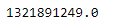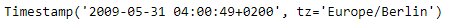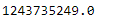# Python | Pandas Timestamp.timestamp

Python is a great language for doing data analysis, primarily because of the fantastic ecosystem of data-centric python packages. Pandas is one of those packages and makes importing and analyzing data much easier.

Pandas` Timestamp.timestamp()` function return the time expressed as the number of seconds that have passed since January 1, 1970. That zero moment is known as the epoch.

Syntax :Timestamp.timestamp()

Parameters : None

Return : number of seconds since zero moment

Example #1: Use `Timestamp.timestamp()` function to return the number of seconds that has passed since the zero moment for the given Timestamp object.

 `# importing pandas as pd ` `import` `pandas as pd ` ` `  `# Create the Timestamp object ` `ts ``=` `pd.Timestamp(year ``=` `2011``,  month ``=` `11``, day ``=` `21``,  ` `                  ``hour ``=` `10``, second ``=` `49``, tz ``=` `'US/Central'``)  ` ` `  `# Print the Timestamp object ` `print``(ts) `

Output :Now we will use the `Timestamp.timestamp()` function to find the number of seconds that has passed.

 `# return the number of seconds ` `ts.timestamp() `

Output :As we can see in the output, the `Timestamp.timestamp()` function has returned a float value indicating this many seconds has passed since the epoch for the given Timestamp object.

Example #2: Use `Timestamp.timestamp()` function to return the number of seconds that has passed since the zero moment for the given Timestamp object.

 `# importing pandas as pd ` `import` `pandas as pd ` ` `  `# Create the Timestamp object ` `ts ``=` `pd.Timestamp(year ``=` `2009``, month ``=` `5``, day ``=` `31``,  ` `                  ``hour ``=` `4``, second ``=` `49``, tz ``=` `'Europe/Berlin'``) ` ` `  `# Print the Timestamp object ` `print``(ts) `

Output :Now we will use the `Timestamp.timestamp()` function to find the number of seconds that has passed.

 `# return the number of seconds ` `ts.timestamp() `

Output :As we can see in the output, the `Timestamp.timestamp()` function has returned a float value indicating this many seconds has passed since the epoch for the given Timestamp object.

My Personal Notes arrow_drop_upCheck out this Author's contributed articles.

If you like GeeksforGeeks and would like to contribute, you can also write an article using contribute.geeksforgeeks.org or mail your article to contribute@geeksforgeeks.org. See your article appearing on the GeeksforGeeks main page and help other Geeks.

Please Improve this article if you find anything incorrect by clicking on the "Improve Article" button below.

Article Tags :

Be the First to upvote.

Please write to us at contribute@geeksforgeeks.org to report any issue with the above content.